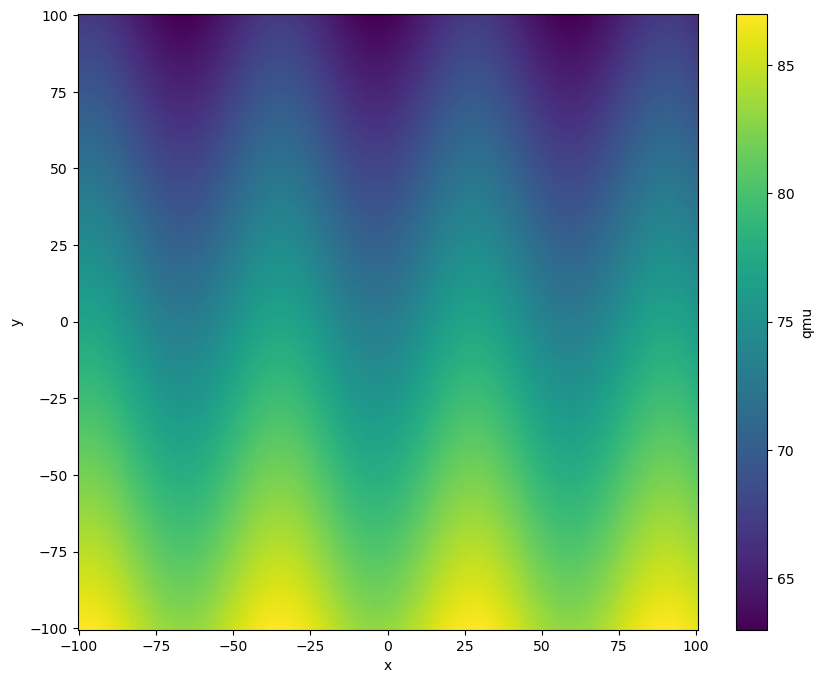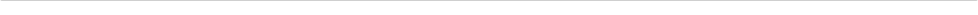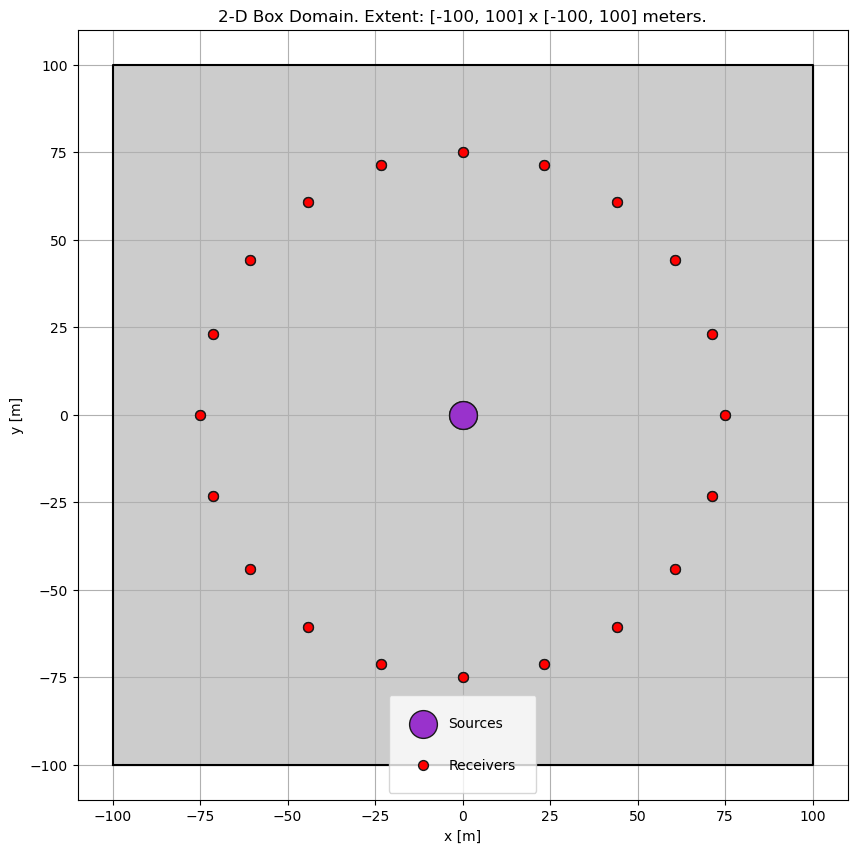Version:

This documentation is not for the latest stable Salvus version.This tutorial is presented as Python code running inside a Jupyter Notebook, the recommended way to use Salvus. To run it yourself you can copy/type each individual cell or directly download the full notebook, including all required files.# Attenuation

This micro-tutorial demonstrates how to run simulations in viscoacoustic / viscoelastic media with SalvusProject. It shows the concept in 2-D but it works just the same in 3-D by just adding an extra dimension.
Copy
import matplotlib.pyplot as plt
import numpy as np
import os
import pathlib
import xarray as xr

import salvus.namespace as sn

# Run simulations on this site.
SALVUS_FLOW_SITE_NAME = os.environ.get("SITE_NAME", "local")

## Parameter Model

There are different ways to define meshes and models in SalvusProject. Here we'll define the multi-parameter model as an xarray data set which will later be interpolated onto our numerical meshes.
# Grid points of the velocity model.
# NOT the grid point of the simulation!
nx, ny = 200, 200

# Create a coordinate grid.
x = np.linspace(-100.0, +100.0, nx)
y = np.linspace(-100.0, +100.0, nx)
xx, yy = np.meshgrid(x, y, indexing="ij")

# Simplistic 5 parameter model with a depth gradient and
# some sinusoidal perturbation.
# This is just an example but this would be the place to add
vp = 1500.0 * np.ones_like(xx) - yy * 0.1 + np.sin(xx / 8 + 0.5) * 2
vs = 1000.0 * np.ones_like(xx) - yy * 0.1 + np.sin(xx / 12 - 0.3) * 2
rho = 980.0 * np.ones_like(xx) - yy * 0.1 + np.sin(xx / 15 + 1) * 2
qkappa = 75.0 * np.ones_like(xx) - yy * 0.1 + np.sin(xx / 6) * 2
qmu = 75.0 * np.ones_like(xx) - yy * 0.1 + np.sin(xx / 10 - 1.2) * 2

# We build a full visco-elastic model with all fields, but will drop
# unneeded fields later on as needed.
dataset = xr.Dataset(
data_vars={
"vp": (["x", "y"], vp),
"vs": (["x", "y"], vs),
"rho": (["x", "y"], rho),
"qkappa": (["x", "y"], qkappa),
"qmu": (["x", "y"], qmu),
},
coords={"x": x, "y": y},
)

# Have a look at the Qmu model.
plt.figure(figsize=(10, 8))
dataset.qmu.T.plot()
plt.show()## Create the Project

# Either load an existing project or create a new one.
p = sn.Project.from_domain(
path="project",
# Should match the volume model.
domain=sn.domain.dim2.BoxDomain(x0=-100, x1=100, y0=-100, y1=100),
)## Simulation Setup

Here we'll create a single explosive source in the center with receivers in a ring around it. The elastic event uses a moment tensor source and records displacement, while the acoustic event requires a scalar source and records phi.
src = sn.simple_config.source.cartesian.MomentTensorPoint2D(
x=0, y=0, mxx=1.0, myy=1.0, mxy=0.0
)

)

src = sn.simple_config.source.cartesian.ScalarPoint2D(x=0, y=0, f=1.0)

)

p.viz.nb.domain()Now we'll add models for acoustic and elastic simulations with and without attenuation. Each scenario uses the full model defined in the dataset above, and we just drop the fields that are not needed.
p += sn.model.volume.cartesian.GenericModel(
name="model_elastic_with_Q", data=dataset
)

# An elastic simulation without attenuation neither requires QMU not QKAPPA
p += sn.model.volume.cartesian.GenericModel(
name="model_elastic_without_Q", data=dataset.drop_vars(["qmu", "qkappa"])
)

# An acoustic simulation without attenuation neither requires Q nor VS
p += sn.model.volume.cartesian.GenericModel(
name="model_acoustic_without_Q",
data=dataset.drop_vars(["qmu", "qkappa", "vs"]),
)

# An acoustic simulation with attenuation neither requires QMU nor VS,
# but requires QKAPPA
p += sn.model.volume.cartesian.GenericModel(
name="model_acoustic_with_Q", data=dataset.drop_vars(["qmu", "vs"])
)
# A few wave-lengths are needed to see an attenuating effect.
center_frequency = 100.0

# Setup four simulations:
# Acoustic / elastic and with or without attenuation.

for medium in ["acoustic", "elastic"]:
for attenuation in [True, False]:
if attenuation:
simulation_name = f"{medium}_with_attenuation"
mc = sn.ModelConfiguration(
background_model=None,
volume_models=f"model_{medium}_with_Q",
# Attenuation results in slightly frequency dependent wave speed.
# Thus one must specify at what frequency they are specified.
# They are often given at 1 Hz.
linear_solids=sn.LinearSolids(reference_frequency=1.0),
)
else:
simulation_name = f"{medium}_without_attenuation"
mc = sn.ModelConfiguration(
background_model=None,
volume_models=f"model_{medium}_without_Q",
)

p += sn.SimulationConfiguration(
name=simulation_name,
max_frequency_in_hertz=center_frequency * 2.0,
elements_per_wavelength=2.0,
model_configuration=mc,
event_configuration=sn.EventConfiguration(
wavelet=sn.simple_config.stf.Ricker(
center_frequency=center_frequency
),
waveform_simulation_configuration=sn.WaveformSimulationConfiguration(
end_time_in_seconds=0.07,
# This is to tell the simulation to actually
# simulate with attenuation or not.
attenuation=attenuation,
),
),
)
As an example, we visualize the simulation setup for the viscoelastic simulation.
p.viz.nb.simulation_setup("elastic_with_attenuation")
[2023-07-03 12:15:19,436] INFO: Creating mesh. Hang on.
<class 'salvus.project.configuration.model.background.homogeneous.IsotropicElastic'>